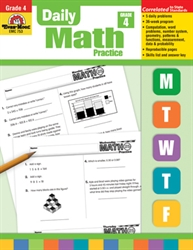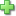# Daily Math Practice Grade 4

Price: \$22.99

See series description for full review.

The skills practiced in Daily Math Practice, Grade 4 include:

Computation

• multidigit addition with and without regrouping
• multidigit subtraction with and without regrouping
• multidigit multiplication
• division with single-digit divisor
• addition and subtraction of fractions

Numbers

• base ten system
• word/standard forms
• place value
• rounding
• odd/even numbers
• ordinals
• estimation
• and many more

Patterns/ Algebra

• figural patterns
• numerical patterns
• expressions
• function tables
• ratios

Geometry/Spatial

• 2-dimentional shapes
• 3-dimentional shapes
• symmetry
• and much more

Measurement

• weight
• capacity
• time
• temperature
• length
• and much more

Data/Probability

• coordinate graphing
• number lines
• constructing graphs
• interpreting graphs
• range
• mode
• median
• probability
• permutations/combinations
Did you find this review helpful?
Series Description
Related Categories
Recommended for...
Customer ReviewsClick here to write a review